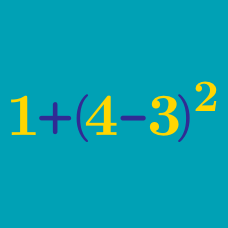Basic Mathematics

Number Line Warmup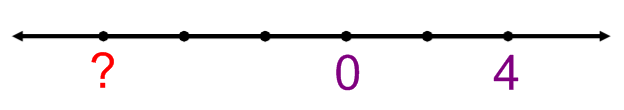What number goes in the space marked by a question mark?

(NOTE: Not all number lines have the distance between points be a unit of 1; any scale is possible.)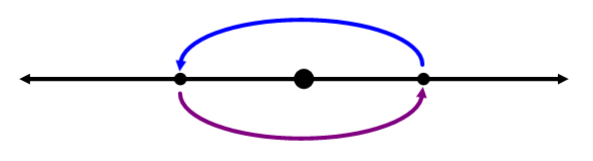The number line above shows an operation that is repeating. What could the operation be?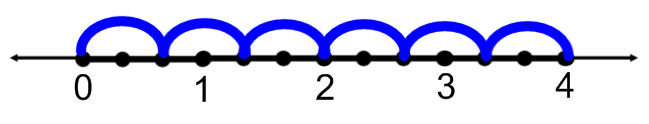What does the picture above illustrate?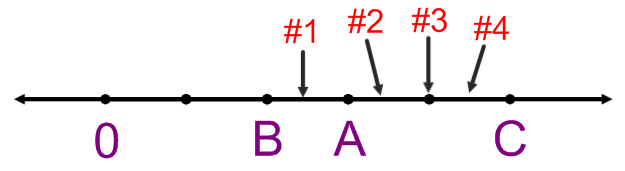If

$\frac{A}{B} = \frac{C}{D}$

then where is D?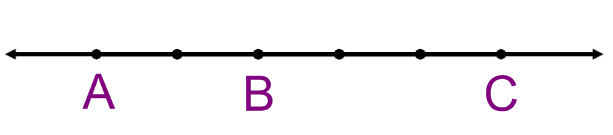Which of these could be the values of $A$, $B$, and $C$?

×